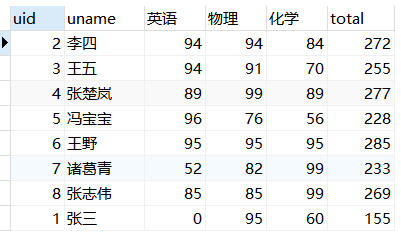﻿ MySQL实现行列转换_Mysql_脚本之家
Mysql# MySQL实现行列转换## 1.行转列的几种方法

### 1.1 case... when ... then ... else ... end

```select uname,uid, -- 正常查询的字段
sum(
case
when course ='英语' then score -- 需要转换的字段
else 0
end) '英语',
sum(
case
when course= '物理' then score
else 0
end) '物理',
sum(
case
when course='化学' then score
else 0
end) '化学'
from course
group by uid```

```case course
when '化学' then score
else 0
end```

### 1.2 if (`字段名1`=‘字段值’，，)

```select uname,uid,
sum(if(`course`='英语',score,0)) '英语',
sum(if(`course`='物理',score,0)) '物理',
sum(if(`course`='化学',score,0)) '化学'
from course
group by uname```total这一列简单，直接在之前的查询基础上加一个sum(score) 'total'即可；Total这一行则可以看成之前的查询不加group by而聚合成一行。因此可以看做是两个表组合到一起如图4和图5：### 1.3 if (`字段名1`=‘字段值’，，) + union

```select uid,uname,
sum(if(`course`='英语',score,0)) '英语',
sum(if(`course`='物理',score,0)) '物理',
sum(if(`course`='化学',score,0)) '化学',
sum(score) 'total'
from course
group by uname
union
select 'Total',null,
sum(if(`course`='英语',score,0)) '英语',
sum(if(`course`='物理',score,0)) '物理',
sum(if(`course`='化学',score,0)) '化学',
sum(score) 'total'
from course```

### 1.4 if (`字段名1`=‘字段值’，，) + IFNULL（）+with rollup

```select ifnull(uid,'Total') uid, uname,
sum(if(`course`='英语',score,0)) '英语',
sum(if(`course`='物理',score,0)) '物理',
sum(if(`course`='化学',score,0)) '化学',
sum(score) 'total'
from course
group by uid
with ROLLUP```## 2.列转行```create view rtc as
select ifnull(uid,'Total') uid,uname,
sum(if(`course`='英语',score,0)) '英语',
sum(if(`course`='物理',score,0)) '物理',
sum(if(`course`='化学',score,0)) '化学',
sum(score) 'total'
from course
group by uid
with ROLLUP
-- 下面是列转行代码
select uid,uname,'英语' course,英语 score from rtc where uid <>'Total' and 英语>0
union all select uid,uname,'物理' ,物理 from rtc where uid <>'Total' and 物理>0
union all select uid,uname,'化学' ,化学 from rtc where uid <>'Total' and 化学>0```